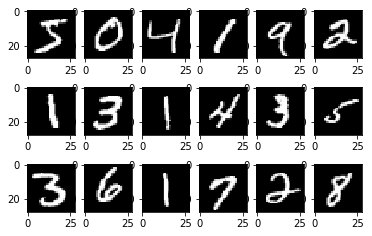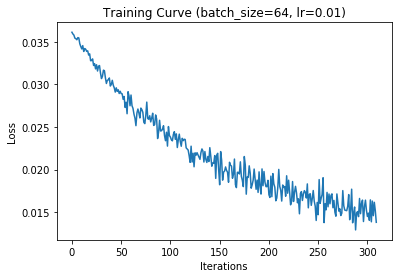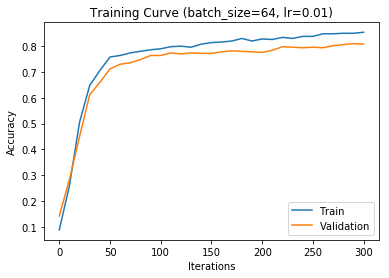# CSC321 Tutorial 4: Multi-Class Classification with PyTorch¶

In this tutorial, we'll go through an example of a multi-class linear classification problem using PyTorch.

Training models in PyTorch requires much less of the kind of code that you are required to write for project 1. However, PyTorch hides a lot of details of the computation, both of the computation of the prediction, and the computation of the gradients. In your later projects, you'll work with both numpy to understand deeply how your models actually work, but also learn PyTorch to gain practical skills in building machine learning models.

In the process, we will:

• Introduce the MNIST dataset, which contains 28x28 pixel images of hand-written digits
• Introduce how to use of PyTorch to build and train models
• (If we have time) explore the effect of certain settings on our model:
• Data set size
• Batch size
• Regularization
In :
%matplotlib inline


## Data¶

The MNIST dataset contains black and white, hand-written (numerical) digits that are 28x28 pixels large. This is a data set that is typically used for demonstrations of machine learning models, and as a first data set to test new types of models.

We will download the dataset. For simplicity, we'll only use the first 2500 images in the MNIST dataset. The first time you run this code, we will download the MNIST dataset.

In :
from torchvision import datasets

mnist_train = list(mnist_train)[:2500]

print(mnist_train)

(<PIL.Image.Image image mode=L size=28x28 at 0x113567BA8>, 5)


Let's take a look at some of the data:

In :
# plot the first 18 images in the training data
import matplotlib.pyplot as plt
for k, (image, label) in enumerate(mnist_train[:18]):
plt.subplot(3, 6, k+1)
plt.imshow(image, cmap='gray')PyTorch has code written for us to convert an image into numerical pixel features. The tensor still preserves the 2D geometry of the image (we still get a 1x28x28 shape) and does not yet flatten the image into a vector (to get a 1x784 shape) like we discussed in lecture.

In :
from torchvision import transforms
# transform the image data type to a 28x28 matrix of numbers
img_to_tensor = transforms.ToTensor()

# convert the last image we saw into a tensor
img_tensor = img_to_tensor(image)
img_tensor.shape

Out:
torch.Size([1, 28, 28])

If we want to convert the entire dataset into these tensor representations (as opposed to PIL.Image objects), there is a transform parameter that we can use when loading the MNIST dataset:

In :
mnist_train = datasets.MNIST('data', train=True, transform=img_to_tensor)
mnist_train = list(mnist_train)[:2500]
print(mnist_train)

(tensor([[[0.0000, 0.0000, 0.0000, 0.0000, 0.0000, 0.0000, 0.0000, 0.0000,
0.0000, 0.0000, 0.0000, 0.0000, 0.0000, 0.0000, 0.0000, 0.0000,
0.0000, 0.0000, 0.0000, 0.0000, 0.0000, 0.0000, 0.0000, 0.0000,
0.0000, 0.0000, 0.0000, 0.0000],
[0.0000, 0.0000, 0.0000, 0.0000, 0.0000, 0.0000, 0.0000, 0.0000,
0.0000, 0.0000, 0.0000, 0.0000, 0.0000, 0.0000, 0.0000, 0.0000,
0.0000, 0.0000, 0.0000, 0.0000, 0.0000, 0.0000, 0.0000, 0.0000,
0.0000, 0.0000, 0.0000, 0.0000],
[0.0000, 0.0000, 0.0000, 0.0000, 0.0000, 0.0000, 0.0000, 0.0000,
0.0000, 0.0000, 0.0000, 0.0000, 0.0000, 0.0000, 0.0000, 0.0000,
0.0000, 0.0000, 0.0000, 0.0000, 0.0000, 0.0000, 0.0000, 0.0000,
0.0000, 0.0000, 0.0000, 0.0000],
[0.0000, 0.0000, 0.0000, 0.0000, 0.0000, 0.0000, 0.0000, 0.0000,
0.0000, 0.0000, 0.0000, 0.0000, 0.0000, 0.0000, 0.0000, 0.0000,
0.0000, 0.0000, 0.0000, 0.0000, 0.0000, 0.0000, 0.0000, 0.0000,
0.0000, 0.0000, 0.0000, 0.0000],
[0.0000, 0.0000, 0.0000, 0.0000, 0.0000, 0.0000, 0.0000, 0.0000,
0.0000, 0.0000, 0.0000, 0.0000, 0.0000, 0.0000, 0.0000, 0.0000,
0.0000, 0.0000, 0.0000, 0.0000, 0.0000, 0.0000, 0.0000, 0.0000,
0.0000, 0.0000, 0.0000, 0.0000],
[0.0000, 0.0000, 0.0000, 0.0000, 0.0000, 0.0000, 0.0000, 0.0000,
0.0000, 0.0000, 0.0000, 0.0000, 0.0118, 0.0706, 0.0706, 0.0706,
0.4941, 0.5333, 0.6863, 0.1020, 0.6510, 1.0000, 0.9686, 0.4980,
0.0000, 0.0000, 0.0000, 0.0000],
[0.0000, 0.0000, 0.0000, 0.0000, 0.0000, 0.0000, 0.0000, 0.0000,
0.1176, 0.1412, 0.3686, 0.6039, 0.6667, 0.9922, 0.9922, 0.9922,
0.9922, 0.9922, 0.8824, 0.6745, 0.9922, 0.9490, 0.7647, 0.2510,
0.0000, 0.0000, 0.0000, 0.0000],
[0.0000, 0.0000, 0.0000, 0.0000, 0.0000, 0.0000, 0.0000, 0.1922,
0.9333, 0.9922, 0.9922, 0.9922, 0.9922, 0.9922, 0.9922, 0.9922,
0.9922, 0.9843, 0.3647, 0.3216, 0.3216, 0.2196, 0.1529, 0.0000,
0.0000, 0.0000, 0.0000, 0.0000],
[0.0000, 0.0000, 0.0000, 0.0000, 0.0000, 0.0000, 0.0000, 0.0706,
0.8588, 0.9922, 0.9922, 0.9922, 0.9922, 0.9922, 0.7765, 0.7137,
0.9686, 0.9451, 0.0000, 0.0000, 0.0000, 0.0000, 0.0000, 0.0000,
0.0000, 0.0000, 0.0000, 0.0000],
[0.0000, 0.0000, 0.0000, 0.0000, 0.0000, 0.0000, 0.0000, 0.0000,
0.3137, 0.6118, 0.4196, 0.9922, 0.9922, 0.8039, 0.0431, 0.0000,
0.1686, 0.6039, 0.0000, 0.0000, 0.0000, 0.0000, 0.0000, 0.0000,
0.0000, 0.0000, 0.0000, 0.0000],
[0.0000, 0.0000, 0.0000, 0.0000, 0.0000, 0.0000, 0.0000, 0.0000,
0.0000, 0.0549, 0.0039, 0.6039, 0.9922, 0.3529, 0.0000, 0.0000,
0.0000, 0.0000, 0.0000, 0.0000, 0.0000, 0.0000, 0.0000, 0.0000,
0.0000, 0.0000, 0.0000, 0.0000],
[0.0000, 0.0000, 0.0000, 0.0000, 0.0000, 0.0000, 0.0000, 0.0000,
0.0000, 0.0000, 0.0000, 0.5451, 0.9922, 0.7451, 0.0078, 0.0000,
0.0000, 0.0000, 0.0000, 0.0000, 0.0000, 0.0000, 0.0000, 0.0000,
0.0000, 0.0000, 0.0000, 0.0000],
[0.0000, 0.0000, 0.0000, 0.0000, 0.0000, 0.0000, 0.0000, 0.0000,
0.0000, 0.0000, 0.0000, 0.0431, 0.7451, 0.9922, 0.2745, 0.0000,
0.0000, 0.0000, 0.0000, 0.0000, 0.0000, 0.0000, 0.0000, 0.0000,
0.0000, 0.0000, 0.0000, 0.0000],
[0.0000, 0.0000, 0.0000, 0.0000, 0.0000, 0.0000, 0.0000, 0.0000,
0.0000, 0.0000, 0.0000, 0.0000, 0.1373, 0.9451, 0.8824, 0.6275,
0.4235, 0.0039, 0.0000, 0.0000, 0.0000, 0.0000, 0.0000, 0.0000,
0.0000, 0.0000, 0.0000, 0.0000],
[0.0000, 0.0000, 0.0000, 0.0000, 0.0000, 0.0000, 0.0000, 0.0000,
0.0000, 0.0000, 0.0000, 0.0000, 0.0000, 0.3176, 0.9412, 0.9922,
0.9922, 0.4667, 0.0980, 0.0000, 0.0000, 0.0000, 0.0000, 0.0000,
0.0000, 0.0000, 0.0000, 0.0000],
[0.0000, 0.0000, 0.0000, 0.0000, 0.0000, 0.0000, 0.0000, 0.0000,
0.0000, 0.0000, 0.0000, 0.0000, 0.0000, 0.0000, 0.1765, 0.7294,
0.9922, 0.9922, 0.5882, 0.1059, 0.0000, 0.0000, 0.0000, 0.0000,
0.0000, 0.0000, 0.0000, 0.0000],
[0.0000, 0.0000, 0.0000, 0.0000, 0.0000, 0.0000, 0.0000, 0.0000,
0.0000, 0.0000, 0.0000, 0.0000, 0.0000, 0.0000, 0.0000, 0.0627,
0.3647, 0.9882, 0.9922, 0.7333, 0.0000, 0.0000, 0.0000, 0.0000,
0.0000, 0.0000, 0.0000, 0.0000],
[0.0000, 0.0000, 0.0000, 0.0000, 0.0000, 0.0000, 0.0000, 0.0000,
0.0000, 0.0000, 0.0000, 0.0000, 0.0000, 0.0000, 0.0000, 0.0000,
0.0000, 0.9765, 0.9922, 0.9765, 0.2510, 0.0000, 0.0000, 0.0000,
0.0000, 0.0000, 0.0000, 0.0000],
[0.0000, 0.0000, 0.0000, 0.0000, 0.0000, 0.0000, 0.0000, 0.0000,
0.0000, 0.0000, 0.0000, 0.0000, 0.0000, 0.0000, 0.1804, 0.5098,
0.7176, 0.9922, 0.9922, 0.8118, 0.0078, 0.0000, 0.0000, 0.0000,
0.0000, 0.0000, 0.0000, 0.0000],
[0.0000, 0.0000, 0.0000, 0.0000, 0.0000, 0.0000, 0.0000, 0.0000,
0.0000, 0.0000, 0.0000, 0.0000, 0.1529, 0.5804, 0.8980, 0.9922,
0.9922, 0.9922, 0.9804, 0.7137, 0.0000, 0.0000, 0.0000, 0.0000,
0.0000, 0.0000, 0.0000, 0.0000],
[0.0000, 0.0000, 0.0000, 0.0000, 0.0000, 0.0000, 0.0000, 0.0000,
0.0000, 0.0000, 0.0941, 0.4471, 0.8667, 0.9922, 0.9922, 0.9922,
0.9922, 0.7882, 0.3059, 0.0000, 0.0000, 0.0000, 0.0000, 0.0000,
0.0000, 0.0000, 0.0000, 0.0000],
[0.0000, 0.0000, 0.0000, 0.0000, 0.0000, 0.0000, 0.0000, 0.0000,
0.0902, 0.2588, 0.8353, 0.9922, 0.9922, 0.9922, 0.9922, 0.7765,
0.3176, 0.0078, 0.0000, 0.0000, 0.0000, 0.0000, 0.0000, 0.0000,
0.0000, 0.0000, 0.0000, 0.0000],
[0.0000, 0.0000, 0.0000, 0.0000, 0.0000, 0.0000, 0.0706, 0.6706,
0.8588, 0.9922, 0.9922, 0.9922, 0.9922, 0.7647, 0.3137, 0.0353,
0.0000, 0.0000, 0.0000, 0.0000, 0.0000, 0.0000, 0.0000, 0.0000,
0.0000, 0.0000, 0.0000, 0.0000],
[0.0000, 0.0000, 0.0000, 0.0000, 0.2157, 0.6745, 0.8863, 0.9922,
0.9922, 0.9922, 0.9922, 0.9569, 0.5216, 0.0431, 0.0000, 0.0000,
0.0000, 0.0000, 0.0000, 0.0000, 0.0000, 0.0000, 0.0000, 0.0000,
0.0000, 0.0000, 0.0000, 0.0000],
[0.0000, 0.0000, 0.0000, 0.0000, 0.5333, 0.9922, 0.9922, 0.9922,
0.8314, 0.5294, 0.5176, 0.0627, 0.0000, 0.0000, 0.0000, 0.0000,
0.0000, 0.0000, 0.0000, 0.0000, 0.0000, 0.0000, 0.0000, 0.0000,
0.0000, 0.0000, 0.0000, 0.0000],
[0.0000, 0.0000, 0.0000, 0.0000, 0.0000, 0.0000, 0.0000, 0.0000,
0.0000, 0.0000, 0.0000, 0.0000, 0.0000, 0.0000, 0.0000, 0.0000,
0.0000, 0.0000, 0.0000, 0.0000, 0.0000, 0.0000, 0.0000, 0.0000,
0.0000, 0.0000, 0.0000, 0.0000],
[0.0000, 0.0000, 0.0000, 0.0000, 0.0000, 0.0000, 0.0000, 0.0000,
0.0000, 0.0000, 0.0000, 0.0000, 0.0000, 0.0000, 0.0000, 0.0000,
0.0000, 0.0000, 0.0000, 0.0000, 0.0000, 0.0000, 0.0000, 0.0000,
0.0000, 0.0000, 0.0000, 0.0000],
[0.0000, 0.0000, 0.0000, 0.0000, 0.0000, 0.0000, 0.0000, 0.0000,
0.0000, 0.0000, 0.0000, 0.0000, 0.0000, 0.0000, 0.0000, 0.0000,
0.0000, 0.0000, 0.0000, 0.0000, 0.0000, 0.0000, 0.0000, 0.0000,
0.0000, 0.0000, 0.0000, 0.0000]]]), 5)


Now, we'll split this data into training and validation, and start to build our model. We won't need a test set for this tutorial, but in general we will also have a test set.

In :
mnist_train, mnist_val = mnist_train[:2000], mnist_train[2000:]


## Linear Model in PyTorch¶

To build a linear model in PyTorch, we create an instance of the class nn.Linear, and specify the number of input features, and the number of output features. For linear regression and binary classification, the number of output features is 1. For multi-class classification, we have as many outputs as there are classes.

When using this model for classification, we'll need to apply the sigmoid or softmax activiation afterwards. That is, this object is only meant to handle the linear part of the model computation.

In :
import torch
import torch.nn as nn

example_model = nn.Linear(50, 1) # assume 50 features, 1 linear output


The example_model object contains weights and biases of the model. By default, PyTorch initializes these values to a random number close to 0:

In :
weight, bias = list(example_model.parameters())
print(weight)
print(weight.shape)
print(bias)
print(bias.shape)

Parameter containing:
tensor([[ 0.0567, -0.0792, -0.0425, -0.0678,  0.0799,  0.0336,  0.0580,  0.0002,
0.1340, -0.0534,  0.0379,  0.1010, -0.1012,  0.1085, -0.1038,  0.0196,
-0.1254, -0.0677, -0.0535, -0.0669,  0.0498,  0.0430, -0.1111, -0.0071,
0.1284, -0.0921,  0.1320,  0.0669, -0.0622, -0.0602, -0.0928, -0.1368,
-0.0660,  0.1033,  0.0281, -0.0570, -0.0748, -0.1127,  0.0222,  0.0861,
0.0167, -0.0192, -0.0235, -0.0236,  0.0063, -0.0910,  0.0763, -0.0043,
torch.Size([1, 50])
Parameter containing:
torch.Size()


If we create a new model, those initial parameters will change:

In :
example_model = nn.Linear(50, 1)
weight, bias = list(example_model.parameters())

# These values should be different from above
print(weight)
print(weight.shape)
print(bias)
print(bias.shape)

Parameter containing:
tensor([[-0.0918, -0.0921,  0.0224, -0.1365, -0.1233,  0.0620, -0.1082, -0.0315,
-0.0448,  0.0789,  0.1304, -0.1239, -0.0810,  0.0469, -0.0680,  0.0409,
-0.0539,  0.0118, -0.1169,  0.0664,  0.0894,  0.0199, -0.1302, -0.1265,
0.1133, -0.0616, -0.1145, -0.0699, -0.0550, -0.0657,  0.1302,  0.0729,
-0.0095,  0.0222, -0.1109,  0.1205,  0.0549, -0.1283,  0.0739,  0.1032,
0.0118, -0.1076,  0.0270,  0.1266, -0.0210,  0.0896, -0.1111, -0.0308,
torch.Size([1, 50])
Parameter containing:
torch.Size()


Now, let's create the actual model that we will train to solve the MNIST digit classification problem. How many input features do we have? How many output features do we need?

In :
model = nn.Linear(784, 10) # 784 = 28*28

# Let's verify that the shapes of the weights and biases are what we expect
weight, bias = list(model.parameters())
print(weight.shape)
print(bias.shape)

torch.Size([10, 784])
torch.Size()


## Making Predictions¶

Let's see how we can make a prediction with this model. (You might find it strange that we're talking about how to make predictions before talking about how to train the model. The reason is that we will always train the model using a varient of gradient descent. So you can imagine that the weights of this model will eventually become more meaningful than it is now)

We'll start with the simpler example_model first. The way that we make predictions is by starting with an input $x$ that has the required shape. Since example_model is just an example, we'll create a tensor with the appropriate shape, filled with random values.

In :
x = torch.randn(50) # create a rank 1 tensor (vector) with 50 features
x.shape

Out:
torch.Size()

To make predictions, we apply the example_model as if it is a function, with the inputs as an argument:

In :
y = example_model(x)
y.shape

Out:
torch.Size()

If this model was used for binary classification, we might also need to apply the sigmoid function:

In :
torch.sigmoid(example_model(x))

Out:
tensor([0.2188], grad_fn=<SigmoidBackward>)

One nice thing about PyTorch is that it vectorizes and parallelizes the computation for us. So, if we had a batch of 32 inputs that we want to make predictions for, we can perform that computation using a single call:

In :
x = torch.randn([32, 50]) # a stack of 32 inputs
print(x.shape)
y = example_model(x)
print(y.shape)

torch.Size([32, 50])
torch.Size([32, 1])


(Note: The order of the dimensions in our input $x$ matters. The batch size always goes first, and the number of features always goes second)

Now, let's try and make some "predictions" with our MNIST model! We still have the variable image_tensor from earlier:

In :
img_tensor.shape

Out:
torch.Size([1, 28, 28])

However, the shape of this tensor is not what we need it to be. We need to flatten the image into either a rank 1 tensor (with shape ) or a rank 2 tensor (with shape [1, 784]). We'll choose the latter, so that the transition to passing multiple images at the same time is easier:

In :
x = img_tensor.view(1, 784)
print(x.shape)
z = model(x)
print(z)
print(z.shape)
y = torch.softmax(z, dim=1)
print(y)

torch.Size([1, 784])
tensor([[-0.2619, -0.2818,  0.1974, -0.1167, -0.1109, -0.2176, -0.2654,  0.1380,
torch.Size([1, 10])
tensor([[0.0821, 0.0805, 0.1300, 0.0950, 0.0955, 0.0859, 0.0819, 0.1225, 0.1027,


The dim=1 in the softmax tells PyTorch which dimension represents different images, and which one represents the different class labels. We want our outputs $y$ to be a probability distribution across the classes, and not the different images.

## Loss Function¶

In order for the network to be useful, we need to actually train it, so that the weights are actually meaningful, non-random values. As we mentioned before, we'll use the network to make predictions, then compare the predictions agains the ground truth via the loss function.

PyTorch has standard loss functions that we can use: for example, nn.BCEWithLogitsLoss() for a binary-classification problem, and a nn.CrossEntropyLoss() for a multi-class classification problem like ours.

In :
criterion = nn.CrossEntropyLoss()


This criterion can also be called as a function. It takes the logit prediction and ground-truth as parameters, and returns the loss. Two things to keep in mind for this function:

1. Loss functions like this usually takes the logit as parameter, rather than the post-softmax probability distributions. This is for numerical stability.
2. This loss function also takes the ground-truth integer index as a parameter, rather than a one-hot vector.
In :
loss = criterion(y, torch.Tensor().long()) # digit 8 = the 8-th class
print(loss)

tensor(2.3000, grad_fn=<NllLossBackward>)


## Optimization and Weight Decay¶

PyTorch also computes derivatives for us using automatic differentiation, which we (might) talk about in this course. In short, we can specify an optimizer (like Stochastic Gradient Descent), and use the optimizer to determine how to update the weights.

In :
import torch.optim as optim
optimizer = optim.SGD(model.parameters(), lr=0.005) # lr = learning rate

# There are three lines of code required to perform
loss.backward()       # compute updates for each parameter
optimizer.step()      # make the updates for each parameter
optimizer.zero_grad() # a clean up step for PyTorch


We can also use weight decay (L2 regularization) in PyTorch through the optimizer:

In :
optimizer = optim.SGD(model.parameters(), lr=0.005, weight_decay=0.01)


## Batching¶

PyTorch data loader also does batching for us!

In :
train_loader = torch.utils.data.DataLoader(mnist_train,
batch_size=32, # batch size
shuffle=True)  # shuffle before each epoch

print(xs) # image pixels
print(ts) # targets
break

# Try changing the batch_size above

0
[tensor([[[[0., 0., 0.,  ..., 0., 0., 0.],
[0., 0., 0.,  ..., 0., 0., 0.],
[0., 0., 0.,  ..., 0., 0., 0.],
...,
[0., 0., 0.,  ..., 0., 0., 0.],
[0., 0., 0.,  ..., 0., 0., 0.],
[0., 0., 0.,  ..., 0., 0., 0.]]],

[[[0., 0., 0.,  ..., 0., 0., 0.],
[0., 0., 0.,  ..., 0., 0., 0.],
[0., 0., 0.,  ..., 0., 0., 0.],
...,
[0., 0., 0.,  ..., 0., 0., 0.],
[0., 0., 0.,  ..., 0., 0., 0.],
[0., 0., 0.,  ..., 0., 0., 0.]]],

[[[0., 0., 0.,  ..., 0., 0., 0.],
[0., 0., 0.,  ..., 0., 0., 0.],
[0., 0., 0.,  ..., 0., 0., 0.],
...,
[0., 0., 0.,  ..., 0., 0., 0.],
[0., 0., 0.,  ..., 0., 0., 0.],
[0., 0., 0.,  ..., 0., 0., 0.]]],

...,

[[[0., 0., 0.,  ..., 0., 0., 0.],
[0., 0., 0.,  ..., 0., 0., 0.],
[0., 0., 0.,  ..., 0., 0., 0.],
...,
[0., 0., 0.,  ..., 0., 0., 0.],
[0., 0., 0.,  ..., 0., 0., 0.],
[0., 0., 0.,  ..., 0., 0., 0.]]],

[[[0., 0., 0.,  ..., 0., 0., 0.],
[0., 0., 0.,  ..., 0., 0., 0.],
[0., 0., 0.,  ..., 0., 0., 0.],
...,
[0., 0., 0.,  ..., 0., 0., 0.],
[0., 0., 0.,  ..., 0., 0., 0.],
[0., 0., 0.,  ..., 0., 0., 0.]]],

[[[0., 0., 0.,  ..., 0., 0., 0.],
[0., 0., 0.,  ..., 0., 0., 0.],
[0., 0., 0.,  ..., 0., 0., 0.],
...,
[0., 0., 0.,  ..., 0., 0., 0.],
[0., 0., 0.,  ..., 0., 0., 0.],
[0., 0., 0.,  ..., 0., 0., 0.]]]]), tensor([8, 4, 0, 4, 3, 7, 9, 4, 2, 4, 9, 3, 9, 2, 8, 7, 6, 0, 5, 7, 6, 0, 3, 5,
0, 0, 6, 2, 3, 3, 7, 2])]


## Putting it all together...¶

In :
def run_gradient_descent(model,
batch_size=64,
learning_rate=0.01,
weight_decay=0,
num_epochs=10):
criterion = nn.CrossEntropyLoss()
optimizer = optim.SGD(model.parameters(), lr=learning_rate, weight_decay=weight_decay)

iters, losses = [], []
iters_sub, train_acc, val_acc  = [], [] ,[]

mnist_train,
batch_size=batch_size,
shuffle=True)

# training
n = 0 # the number of iterations
for epoch in range(num_epochs):
if len(ts) != batch_size:
continue
xs = xs.view(-1, 784)    # flatten the image. The -1 is a wildcard
zs = model(xs)
loss = criterion(zs, ts) # compute the total loss
loss.backward()          # compute updates for each parameter
optimizer.step()         # make the updates for each parameter
optimizer.zero_grad()    # a clean up step for PyTorch

# save the current training information
iters.append(n)
losses.append(float(loss)/batch_size)  # compute *average* loss

if n % 10 == 0:
iters_sub.append(n)
train_acc.append(get_accuracy(model, mnist_train))
val_acc.append(get_accuracy(model, mnist_val))
# increment the iteration number
n += 1

# plotting
plt.title("Training Curve (batch_size={}, lr={})".format(batch_size, learning_rate))
plt.plot(iters, losses, label="Train")
plt.xlabel("Iterations")
plt.ylabel("Loss")
plt.show()

plt.title("Training Curve (batch_size={}, lr={})".format(batch_size, learning_rate))
plt.plot(iters_sub, train_acc, label="Train")
plt.plot(iters_sub, val_acc, label="Validation")
plt.xlabel("Iterations")
plt.ylabel("Accuracy")
plt.legend(loc='best')
plt.show()

return model

def get_accuracy(model, data):

correct, total = 0, 0
xs = xs.view(-1, 784) # flatten the image
zs = model(xs)
pred = zs.max(1, keepdim=True) # get the index of the max logit
correct += pred.eq(ts.view_as(pred)).sum().item()
total += int(ts.shape)
return correct / total


Let's try training this model!

In :
model = nn.Linear(784, 10)Linear(in_features=784, out_features=10, bias=True)